xwvd

Cross Wigner-Ville distribution and cross smoothed pseudo Wigner-Ville distribution

Description

example

d = xwvd(x,y) returns the cross Wigner-Ville distribution of x and y.

example

d = xwvd(x,y,fs) returns the cross Wigner-Ville distribution when x and y are sampled at a rate fs.

d = xwvd(x,y,ts) returns the cross Wigner-Ville distribution when x and y are sampled with a time interval ts between samples.

d = xwvd(___,'smoothedPseudo') returns the cross smoothed pseudo Wigner-Ville distribution of x and y. The function uses the length of the input signals to choose the lengths of the windows used for time and frequency smoothing. This syntax can include any combination of input arguments from previous syntaxes.

d = xwvd(___,'smoothedPseudo',twin,fwin) specifies the time window, twin, and the frequency window, fwin, used for smoothing. To use the default window for either time or frequency smoothing, specify the corresponding argument as empty, [].

d = xwvd(___,'smoothedPseudo','NumFrequencyPoints',nf) computes the cross smoothed pseudo Wigner-Ville distribution using nf frequency points. You can specify twin and fwin in this syntax, or you can omit them.

d = xwvd(___,'MinThreshold',thresh) sets to zero those elements of d whose amplitude is less than thresh. This syntax applies to both the cross Wigner-Ville distribution and the cross smoothed pseudo Wigner-Ville distribution.

[d,f,t] = xwvd(___) also returns a vector of frequencies, f, and a vector of times, t, at which d is computed.

example

xwvd(___) with no output arguments plots the real part of the cross Wigner-Ville or cross smoothed pseudo Wigner-Ville distribution in the current figure.

Examples

collapse all

Generate two signals sampled at 1 kHz for 1 second and embedded in white noise. One signal is a sinusoid of frequency 150 Hz. The other signal is a chirp whose frequency varies sinusoidally between 200 Hz and 400 Hz. The noise has a variance of $0.{1}^{2}$.

fs = 1000;
t = (0:1/fs:1)';

x = cos(2*pi*t*150) + 0.1*randn(size(t));
y = vco(cos(3*pi*t),[200 400],fs) + 0.1*randn(size(t));

Compute the Wigner-Ville distribution of the sum of the signals.

wvd(x+y,fs)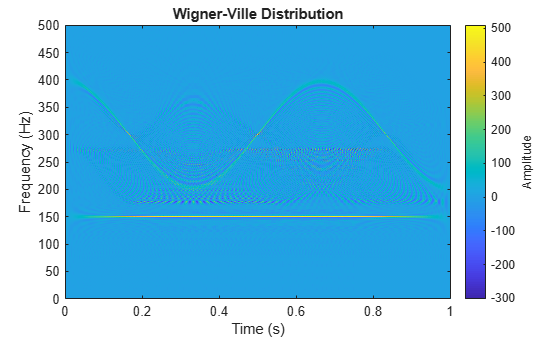Compute and plot the cross Wigner-Ville distribution of the signals. The cross-distribution corresponds to the cross-terms of the Wigner-Ville distribution.

xwvd(x,y,fs)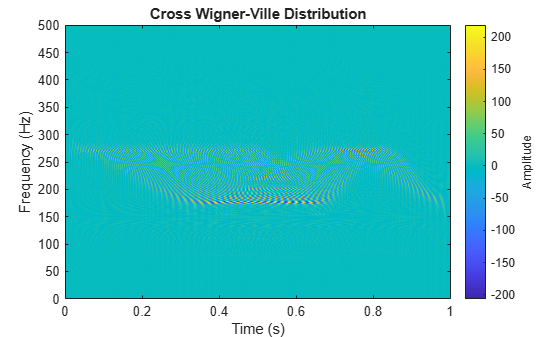Generate a two-channel signal that consists of two chirps. The signal is sampled at 3 kHz for one second. The first chirp has an initial frequency of 400 Hz and reaches 800 Hz at the end of the sampling. The second chirp starts at 500 Hz and reaches 1000 Hz at the end. The second chirp has twice the amplitude of the first chirp.

fs = 3000;
t = (0:1/fs:1-1/fs)';

x1 = chirp(t,1400,t(end),800);
x2 = 2*chirp(t,200,t(end),1000);

Store the signal as a timetable. Compute and plot the cross Wigner-Ville distribution of the two channels.

xt = timetable(seconds(t),x1,x2);

xwvd(xt(:,1),xt(:,2))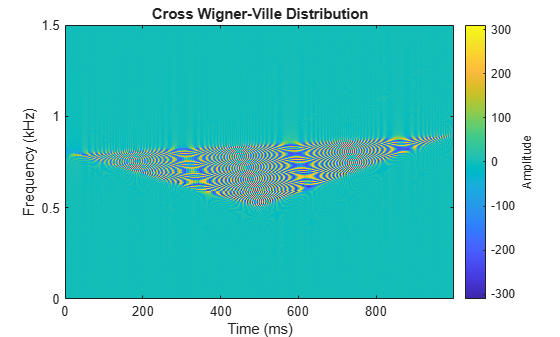Compute the instantaneous frequency of a signal by using a known reference signal and the cross Wigner-Ville distribution.

Create a reference signal consisting of a Gaussian atom sampled at 1 kHz for 1 second. A Gaussian atom is a sinusoid modulated by a Gaussian. Specify a sinusoid frequency of 50 Hz. The Gaussian is centered at 64 milliseconds and has a variance of $0.{01}^{2}$.

fs = 1e3;
t = (0:1/fs:1-1/fs)';

mu = 0.064;
sigma = 0.01;
fsin = 50;

xr = exp(-(t-mu).^2/(2*sigma^2)).*sin(2*pi*fsin*t);

Create the "unknown" signal to analyze, consisting of a chirp. The signal starts suddenly at 0.4 second and ends suddenly half a second later. In that lapse, the frequency of the chirp decreases linearly from 400 Hz to 100 Hz.

f0 = 400;
f1 = 100;

xa = zeros(size(t));
xa(t>0.4 & t<=0.9) = chirp((0:1/fs:0.5-1/fs)',f0,0.5,f1);

Create a two-component signal consisting of the sum of the unknown and reference signals. The smoothed pseudo Wigner-Ville distribution of the result provides an "ideal" time-frequency representation.

Compute and display the smoothed pseudo Wigner-Ville distribution.

w = wvd(xa+xr,fs,'smoothedPseudo');

wvd(xa+xr,fs,'smoothedPseudo')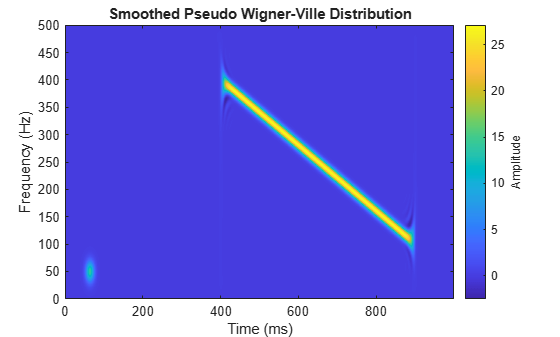Compute the cross Wigner-Ville distribution of the unknown and reference signals. Take the absolute value of the distribution and set to zero the elements with amplitude less than 10. The cross Wigner-Ville distribution is equal to the cross-terms of the two-component signal.

Plot the real part of the cross Wigner-Ville distribution.

[c,fc,tc] = xwvd(xa,xr,fs);
c = abs(c);
c(c<10) = 0;

xwvd(xa,xr,fs)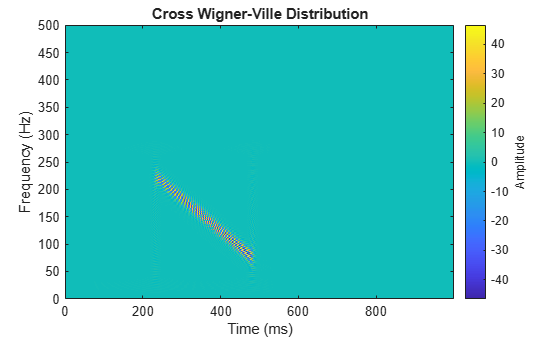Enhance the Wigner-Ville cross-terms by adding the ideal time-frequency representation to the cross Wigner-Ville distribution. The cross-terms of the Wigner-Ville distribution occur halfway between the reference signal and the unknown signal.

d = w + c;

d = abs(real(d));

imagesc(tc,fc,d)
axis xy
colorbar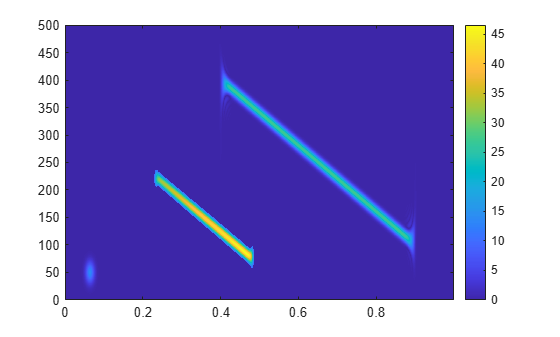Identify and plot the high-energy ridge corresponding to the cross-terms. To isolate the ridge, find the time values where the cross-distribution has nonzero energy.

ff = tfridge(c,fc);

tv = sum(c)>0;

ff = ff(tv);
tc = tc(tv);

hold on
plot(tc,ff,'r--','linewidth',2)
hold off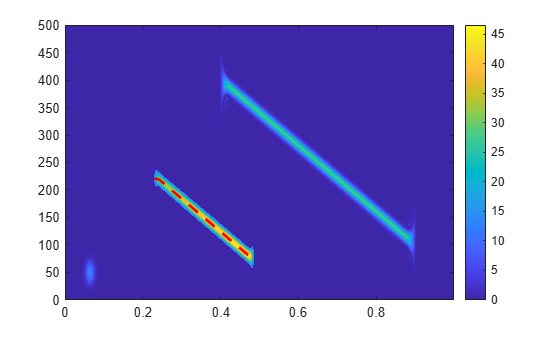Reconstruct the instantaneous frequency of the unknown signal by using the ridge and the reference function. Plot the instantaneous frequency as a function of time.

tEst = 2*tc - mu;
fEst = 2*ff - fsin;

plot(tEst,fEst)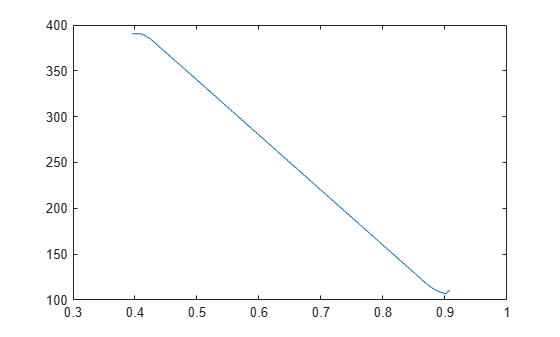Input Arguments

collapse all

Input signals, specified as vectors or MATLAB® timetables each containing a single vector variable. x and y must both be vectors or both be timetables and must have the same length.

If the input signals have odd length, the function appends a zero to make the length even.

Example: cos(pi/8*(0:159))'+randn(160,1)/10 specifies a sinusoid embedded in white noise.

Example: timetable(seconds(0:5)',rand(6,1)) specifies a random variable sampled at 1 Hz for 4 seconds.

Data Types: single | double
Complex Number Support: Yes

Sample rate, specified as a positive numeric scalar.

Sample time, specified as a duration scalar.

Time and frequency windows used for smoothing, specified as vectors of odd length. By default, xwvd uses Kaiser windows with shape factor β = 20.

• The default length of twin is the smallest odd integer greater than or equal to round(length(x)/10).

• The default length of fwin is the smallest odd integer greater than or equal to nf/4.

Each window must have a length smaller than or equal to 2*ceil(length(x)/2).

Example: kaiser(65,0.5) specifies a 65-sample Kaiser window with a shape factor of 0.5.

Number of frequency points, specified as an integer. This argument controls the degree of oversampling in frequency. The number of frequency points must be at least (length(fwin)+1)/2 and cannot be greater than the default.

Minimum nonzero value, specified as a real scalar. The function sets to zero those elements of d whose amplitudes are less than thresh.

Output Arguments

collapse all

Cross Wigner-Ville distribution, returned as a matrix. Time increases across the columns of d, and frequency increases down the rows. The matrix is of size Nf × Nt, where Nf is the length of f and Nt is the length of t.

Frequencies, returned as a vector.

• If the input has time information, then f contains frequencies expressed in Hz.

• If the input does not have time information, then f contains normalized frequencies expressed in rad/sample.

Time instants, returned as a vector.

• If the input has time information, then t contains time values expressed in seconds.

• If the input does not have time information, then t contains sample numbers.

The number of time points is fixed as 4*ceil(length(x)/2).

collapse all

Cross Wigner-Ville Distribution

For continuous signals x(t) and y(t), the cross Wigner-Ville distribution is defined as

${\mathrm{XWVD}}_{x,y}\left(t,f\right)={\int }_{-\infty }^{\infty }x\left(t+\frac{\tau }{2}\right){y}^{*}\left(t-\frac{\tau }{2}\right){e}^{-j2\pi f\tau }\text{\hspace{0.17em}}d\tau .$

For a discrete signal with N samples, the distribution becomes

${\text{XWVD}}_{x,y}\left(n,k\right)=\sum _{m=-N}^{N}x\left(n+m/2\right)\text{\hspace{0.17em}}{y}^{*}\left(n-m/2\right)\text{\hspace{0.17em}}{e}^{-j2\pi km/N}.$

For odd values of m, the definition requires evaluation of the signal at half-integer sample values. It therefore requires interpolation, which makes it necessary to zero-pad the discrete Fourier transform to avoid aliasing.

The cross Wigner-Ville distribution contains interference terms that often complicate its interpretation. To sharpen the distribution, one can filter the definition with lowpass windows. The cross smoothed pseudo Wigner-Ville distribution uses independent windows to smooth in time and frequency:

${\text{XSPWVD}}_{x,y}^{g,H}\left(t,f\right)={\int }_{-\infty }^{\infty }g\left(t\right)\text{\hspace{0.17em}}H\left(f\right)\text{\hspace{0.17em}}x\left(t+\frac{\tau }{2}\right){y}^{*}\left(t-\frac{\tau }{2}\right){e}^{-j2\pi f\tau }\text{\hspace{0.17em}}d\tau .$

 Cohen, Leon. Time-Frequency Analysis: Theory and Applications. Englewood Cliffs, NJ: Prentice-Hall, 1995.

 Mallat, Stéphane. A Wavelet Tour of Signal Processing. Second Edition. San Diego, CA: Academic Press, 1999.

 Malnar, Damir, Victor Sucic, and Boualem Boashash. "A cross-terms geometry based method for components instantaneous frequency estimation using the cross Wigner-Ville distribution." In 11th International Conference on Information Sciences, Signal Processing and their Applications (ISSPA), pp. 1217–1222. Montréal: IEEE®, 2012.